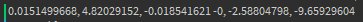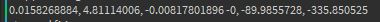# Increasing/decreasing the direction of ray on the Y axis

Hello! Recently I made a gun with camera recoil, and I want to switch it so instead of the camera going up, the bullets will go up.

I am currently using Ray.new for the bullet. X is a number that changes from 1 to 20.

``````local ray = Ray.new(Gun.ShootFrom.Position, (cameraCFrame.lookVector * cameraCFrame.Y * x) * (10))
``````

I tried looking for a solution, and also tried changing the code (not really knowledge-able of CFrames) with no success.

How can I increase/decrease the direction of the ray on the Y axis?

• -Vector3.new(0,y,0)

Use Vector3 maths. Change the y value to a number.

If X is meant to be in degrees of the angle change you could use:

`(cameraCFrame * CFrame.Angles(0, 0, math.rad(x))).LookVector`

So

``````local ray = Ray.new(Gun.ShootFrom.Position, (cameraCFrame * CFrame.Angles(0, 0, math.rad(x))).LookVector * (10))
``````

Thanks for the replies, but both of the methods doesn’t cast the ray to the right target.

Using (cameraCFrame * CFrame.Angles(0, 0, math.rad(x))).LookVector[initial] Using (cameraCFrame.lookVector * cameraCFrame.Y * x) * (10)) - cast the right way, but doesn’t change YCFrame.Y is just the Y component of the CFrame’s position vector. You are multiplying it by the LookVector which is the forward-facing unit vector of the CFrame, so you wouldn’t be changing the direction at all; it’s just making the ray longer.

Oh, alright, thanks for the information, but does that include the cameraCFrame * CFrame.Angles(0, 0, math.rad(x))).LookVector or only the cameraCFrame.lookVector * cameraCFrame.Y * x?

I still didn’t figure out how to change the Y target.